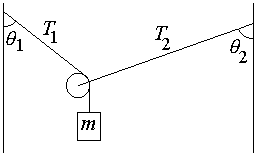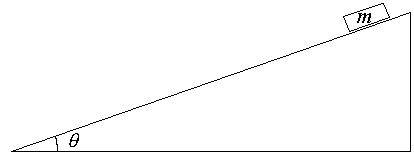First Name: ___________________ Last Name: ____________________ Section: _________

February 20, 1998 Physics 103

Exam 1

Print your name and section clearly on all five pages. (If you do not know your section number, write your TAs name.) Show all work in the space immediately below each problem. Your final answer must be placed in the box provided. Problems will be graded on reasoning and intermediate steps as well as on the final answer. Be sure to include units wherever necessary, and the direction of vectors. Each problem is worth 25 points. In doing the problems, try to be neat. Check your answers to see that they have the correct dimensions (units) and are the right order of magnitudes. You are allowed one 8½ x 11" sheet of notes and no other references. The exam lasts exactly 50 minutes.

(Do not write below)

SCORE:

Problem 1: __________

Problem 2: __________

Problem 3: __________

Problem 4: __________

TOTAL: ___________

First Name: ___________________ Last Name: ____________________ Section: _________

1. A car with a mass of m = 1000 kg is traveling down a long straight road at a constant speed of 25 m/s. At t = 0 the brakes are applied, and the car skids to rest with a constant deceleration of a = - 5 m/s2.

a. What is the speed of the car at t = 3 seconds? (5 pts.)

b. At what time does the car come to rest? (5 pts.)

c. How far does the car travel before coming to rest? (5 pts.)

d. What is the friction force between the tires and the road? (5 pts.)

e. What is the coefficient of kinetic friction between the tires and the road? (5 pts.)

First Name: ___________________ Last Name: ____________________ Section: _________

2. A ball is thrown at t = 0 with an initial speed of 20 m/s at an angle of 45 degrees above the horizontal.

a. What maximum height does the ball reach? (5 pts.)

b. At what time does the ball reach its maximum height? (5 pts.)

c. What is the velocity of the ball (magnitude and direction) when it reaches its maximum height? (5 pts.)

d. How far does the ball travel horizontally before it returns to its original height? (5 pts.)

e. What is the velocity of the ball (magnitude and direction) when it returns to its original height? (5 pts.)

First Name: ___________________ Last Name: ____________________ Section: _________

3. A 5-kg mass is suspended by two strings and by a massless pulley as shown. The angle q1 is 30 degrees.1. Calculate the tension T1. (5 pts.)
2.

3. Calculate the angle q2. (10 pts.)

c. Calculate the tension T2. (10 pts.)

First Name: ___________________ Last Name: ____________________ Section: _________

4. A block is released from rest and slides down a plane inclined at an angle of q = 20 degrees as shown. The coefficient of kinetic friction between the block and the plane is m = 0.2.a. Calculate the acceleration of the block. (10 pts.)

b. How long does it take for the block to slide 50 cm along the plane? (5 pts.)

c. What speed does the block have after it has slid 50 cm along the plane? (5 pts.)

d. What coefficient of static friction is required to keep the block from slipping? (5 pts.)